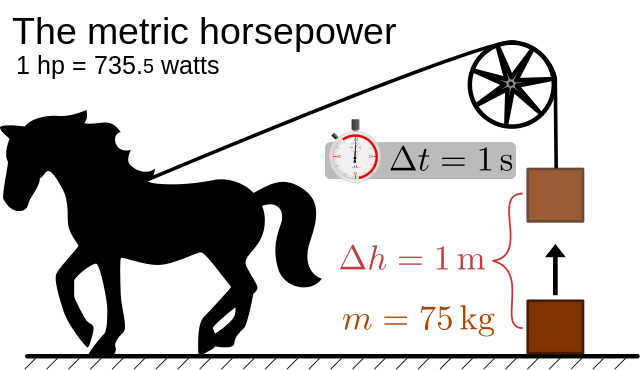#Difference Between Similar Terms and Objects

# Difference Between Ohms And WattsWe need electricity for making our lives comfortable and productive, but do all know

how it is measured. We say ohms, watts, volts and amps. But what are they? Though

we buy a 40-watt bulb, 240 volts UPS, or 8 ohm speakers, many of us don’t try to know

what the terms denote. Understanding these terms and their relationship will teach us

##Ohm means Resistance

Resistance is the property of a material to resist an electrical current flowing through it.

The measuring unit of this resistance is called the ohm which is represented as the

omega sign, Ω. It is named after the German physicist Georg Simon Ohm. The Ohm’s

Law is expressed as I = V / R, where I is electrical current (Amperes), V is the Voltage

and R is Resistance (Ohms). That means a current is equal to the voltage divided by

the resistance. Ohm is the standard unit of electrical resistance as per the International

System of Units, and it also stands for the reactance in Alternating Current and Radio

frequency applications. An ohm is equivalent to a volt per ampere. The unit of

conductance is called “mho” which is a reverse spelling of the word “ohm”.

The ohm is defined as the resistance between two terminals of a conducting material

when a constant potential difference of 1.0 volts is applied to produce 1.0 ampere

current in the conducting material, provided there is no electromotive force. The flow of

electrons through a material is directly proportional to its pressure on the electrons and

its cross sectional area.

All electrical circuits will have a voltage source, either as Direct current (DC) like a

battery or an Alternating Current (AC), such as load. When connected, the current (A)

flows from the negative terminal to the positive terminal along the wire. During this

process, a resistance against the flow of the current is generated in the wire, which is

measured as ohm. And the quantity of power developed subsequent to the resistance,

either in the form of light or in the form of heat, is measured as Watt (W). An ampere

(amp) is the amount of current in a circuit, whereas, watt is the quantity of electrical

power generated by the circuit per second.

To explain an ohm, further, let’s consider the working of a speaker. When the electricity

flows into the speaker, there occurs some resistance against the flow of current in the

speaker. The ohms rating of the speaker depends on how much electricity it resists, and

how much energy it uses. If the ohm rating is high, the performance of the speaker will

be low. The ohms and watts are used to know the quantity of each while handling them.

A watt is the SI unit of power, and is named after the Scottish inventor, James Watt,

who lived during the period: 1736–1819. One watt is the quantity of work done when

one amp of current flows through a conductor having electrical potential difference of

one volt. In other words, a watt is one joule of energy used per second; where joule

denotes energy, and watt, the power. That is, a watt is one Joule per second or

energy/time. Therefore, it is both the energy spent, and the time to taken to expend it.

A kilowatt is a thousand  watts, which is equal to 1.34 horsepower. The output power of engines, electric motors, heaters etc., is expressed in kilowatts. The amount of

electromagnetic power output of radio and television transmitters is also calculated in

kilowatts. It is found that on a sunny day, the earth’s equatorial regions receive

approximately 1 kilowatt sunlight per 1sq. meter surface area.

Latest posts by Punalur Chandrasekaran (see all)

### Search DifferenceBetween.net :

Custom Search

Help us improve. Rate this post!Loading...Email This Post : If you like this article or our site. Please spread the word. Share it with your friends/family.

1. thanks am greatfull

2. I am quoting this ” watt is one Joule per second or energy/time” to mention a scientific error: “or” sends the message of one or the other. They are not. Magnitudes and they are units are realted but different concepts.

Please note: comment moderation is enabled and may delay your comment. There is no need to resubmit your comment.

## References :

http://serc.carleton.edu/integrate/teaching_materials/energy_sustain/student_materials/

http://www.nde- ed.org/EducationResources/CommunityCollege/EddyCurrents/Physics/currentflow.htm